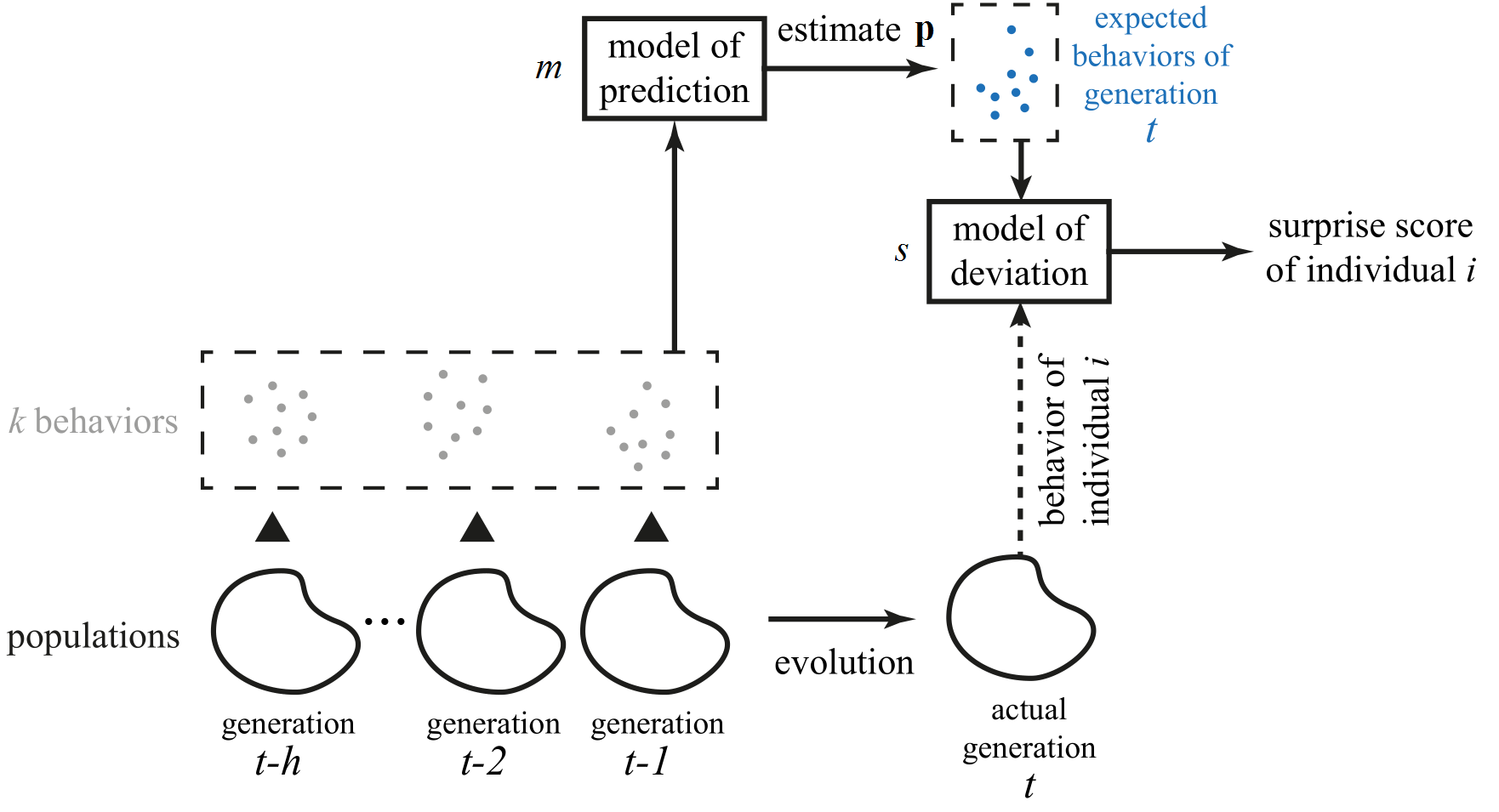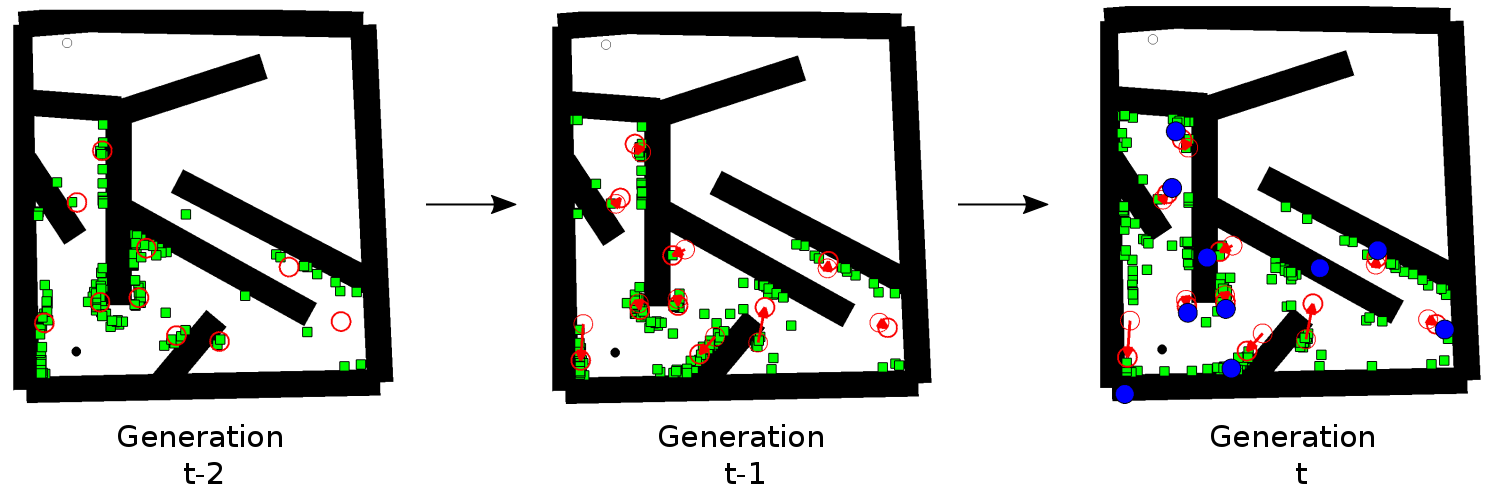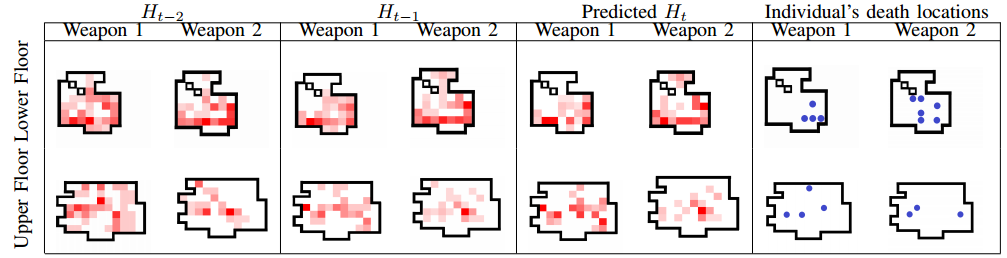# Surprise Search

Surprise search is a novel algorithm that takes inspiration from the notion of surprise for unconventional discovery in computational creativity.

The algorithm mimics the self-surprise cognitive process of creativity and equips computational creators with the ability to search for outcomes that deviate from the algorithm’s expected behavior or process.

The AlgorithmIn order to use surprise as a goal of evolutionary computation, two components are necessary: a predictive model which creates the predictions of future outputs based on past outputs, and a deviation formula which assesses whether (and by which degree) the actual output deviates from the predicted output.

Predictive Model

There is an nigh infinite number of ways in which one can predict future outcomes, from simple interpolation to machine learning. At its core, the vector of predictive outcome p is based on a prediction model (m), the number of past outcomes considered for the prediction (h) and how many predictions are considered per generation (k).

p = m(h, k)

History (h) refers to how far into the past the predictive model observes when making predictions into the future. At the absolute minimum, the two previous generations must be considered, in order to assess a degree of behavioral (outcome) change which can be expected in the current generation. Earlier information can also be used, by looking at previous generations further in the past, or by considering an archive of important past predictions.

Locality (k) refers to the granularity in which the trends of past populations are observed. Locality can stretch from global (i.e. each generation predicts a single descriptive feature of the population of size P in the current generation) to individual (i.e. each individual traces its own lineage of parents, grandparents etc. and attempts to surprise itself). A parameter k determines the level of prediction locality which can vary from 1 (global) to P (individual) or anything inbetween.

Predictive model (m) refers to a model which can calculate a future outcome from current and past data as collected based on k and h. As noted above, any modeling approach can be used for such purposes: from a simple linear regression of points in the outcome space, to non-linear extrapolations, or machine learned models (e.g. artificial neural networks or support vector machines). Depending on the locality of the prediction (k), the model may derive a vector of expected outcomes to deviate from.

Deviation Formula

The deviation formula calculates the surprise score of individual i as the average distance (ds) of the n closest predictions  (pi,j)  made using the predictive model p. This formula assumes that the more divergent an output is from what the expected outcome was, the more surprising it is.Example

Maze NavigationIn the maze navigation task, an agent controlled by an artificial neural network (ANN) enters a maze enclosed by walls at the start position of the maze, and has a specific timeframe to find the goal position of the maze. The ANN is evolved using neuroevolution of augmenting topologies (NEAT) which adds complexity to initially simple networks during the course of evolution.

When using surprise search we suggest grouping individuals into k clusters; each predicted point is calculated based on the linear interpolation of a cluster in the current population and the same cluster in the previous population. In other words, the prediction locality of surprise search in this problem is determined by the number of clusters (k) chosen (k is 10 in this example), the history involves two time slices of the population (two previous generations) and the model is a linear regression function. The  surprise score of individuals in the next generation is calculated based on the deviation (Euclidean distance) of each individual from the closest predicted point. This rewards agents who diverge from an expected improvement in behavior.

The following three videos show the behaviour of three typical runs of surprise search, novelty search, and objective search (for details, see PDF).

Generative ArtIn the generative art example, colorful images are generated via evolving compositional pattern-producing neural networks (CPPNs). These neural networks can have different activation functions (e.g. sigmoid or Gaussian curves) which produce symmetries and repetitions in the output.

Each pixel in the colored image is represented as a HSB (hue, saturation, brightness) triplet, and the CPPN produces these three output values using the x, y coordinates of the pixel as input.

In this example, we predict one expected outcome per individual in the population, taking into account their own parent (i.e. we predict based on genotypic history).

The predicted outcome is based on the differences in HSB values between the parent and grandparent (h = 2) of the evaluated individual, applied on the parent. Similarly to the maze navigation surprise search setup, m is a simple linear regression model and h = 2; however, the k value considered here is P (where P is the size of the population), as each image has an individual prediction.

A surprising outcome, in this example, can be measured based on the per-pixel difference (e.g. ds can be the Euclidean distance) in hue, saturation and brightness between the predicted outcome and the actual offspring.

In the image above, the rightmost image has a high surprise score since the hues of the entire image have shifted to greens and blues, and the image is overall darker.

Game Content GenerationIn this application, surprise search is used to generate surprising content for games. In particular the objective is to generate, under the constraints of balance and efficiency, a surprising sets of weapons for Unreal Tournament III, a first person shooter developed by Epic Games. Therefore a constrained surprise search algorithm is proposed and applied to this problem.

The following videos show some examples of surprising weapons generated through constrained surprise search:

Soft Robots Evolution

This work explores how surprise search can affect the evolution of soft robot morphologies, comparing the performance and the structure of the evolved robots.

Publications

• Daniele Gravina, Antonios Liapis and Georgios N. Yannakakis: “Quality Diversity Through Surprise” arXiv preprint arXiv:1807.02397  (2018). PDF
• Daniele Gravina, Antonios Liapis and Georgios N. Yannakakis: “Fusing Novelty and Surprise for Evolving Robot Morphologies” in Proceedings of the Genetic and Evolutionary Computation Conference . ACM, 2018. PDF
• Daniele Gravina, Antonios Liapis and Georgios N. Yannakakis: “Surprise Search for Evolutionary Divergence” arXiv preprint arXiv:1706.02556 (2017). PDF
• Daniele Gravina, Antonios Liapis and Georgios N. Yannakakis: “Coupling Novelty and Surprise for Evolutionary Divergence” in Proceedings of the Genetic and Evolutionary Computation Conference. ACM, 2017. PDF
• Daniele Gravina, Antonios Liapis and Georgios N. Yannakakis: “Exploring Divergence in Soft Robot Evolution” in Proceedings of the Genetic and Evolutionary Computation Conference Companion. ACM, 2017. PDF
• Daniele Gravina, Antonios Liapis and Georgios N. Yannakakis: “Surprise Search: Beyond Objectives and Novelty” in Proceedings of the Genetic and Evolutionary Computation Conference. ACM, 2016. PDF
• Georgios N. Yannakakis and Antonios Liapis: “Searching for Surprise” in  Proceedings of the International Conference on Computational Creativity, 2016. PDF
• Daniele Gravina, Antonios Liapis and Georgios N. Yannakakis: “Constrained Surprise Search for Content Generation” in Proceedings of the IEEE Conference on Computational Intelligence and Games (CIG), 2016. PDF